[an error occurred while processing this directive]

# Math 896 Section 700 Experimentation, Conjecture and Reasoning Spring 2005### Question of the Day

Using 1 × 1 square tiles and 1 × 2 dominoes, one can tile a 1 × 3 strip of squares three different ways. (Find them!) In how many different ways can one tile a 1 × 4 strip of squares? A 1 × 5 strip? A 1 × 6 strip? Can you generalize? Can you predict how many there are to tile a 1 × 15 strip from your generalization? (I encourage you to build a manipulative for this exercise, even if only using squared graph paper!)### Key Concepts

1. The Fibonacci numbers arise in an astonishing variety of applications and settings as number patterns.
2. Starting from F1 = 1, and F2 = 1 and for n > 2m Fn = Fn-1 + Fn-2, the Fibonacci numbers are 1, 1, 2, 3, 5, 8, 13, 21,... .### Vocabulary

1. Leonardo of Pisa, who wrote under the pen name Fibonacci, may have been the greatest mathematician of the middle ages. His text Liber Abaci introduced the Hindu-Arabic numeral system to Western Europe in 1202. He is now most remembered for a trivial problem in that text counting the number of offspring of a pair of rabbits. Lucas called the sequence of numbers discussed by that problem the Fibonacci numbers. (from Prime Pages Glossary )### Mathematical Ideas

#### Discovering the Fibonacci pattern through spiral counts

If you have a pinecone readily available, count the spirals (in each direction) along the pinecone’s outer surface. If you have a fresh pineapple available, count the spirals on the outer surface of the pineapple. (Then cut and eat the pineapple!) Using the picture of the daisy, count the spirals (each direction) in the daisy center. (It may help to print the picture and draw the spirals!) Using the picture of the sunflower, count the spirals (each direction) in the sunflower. (It will be even better if you have an actual sunflower seed head.)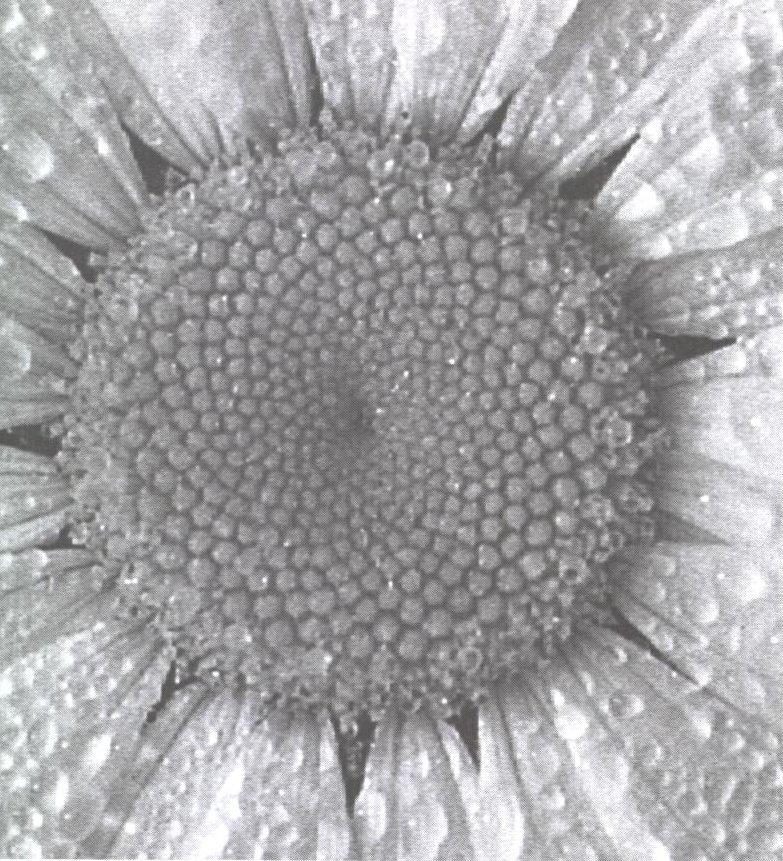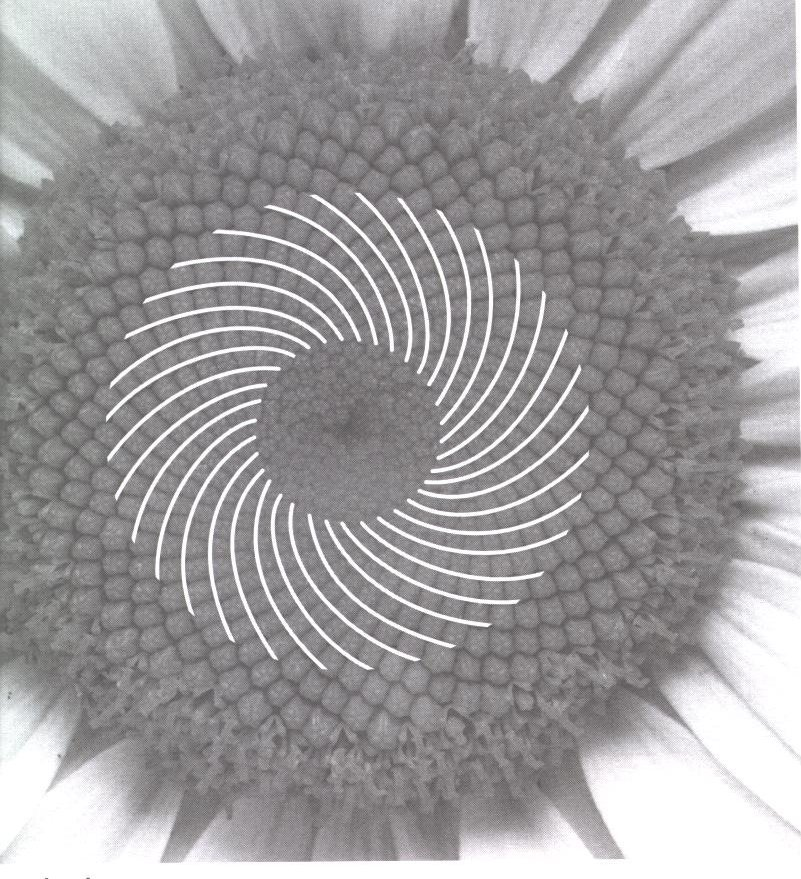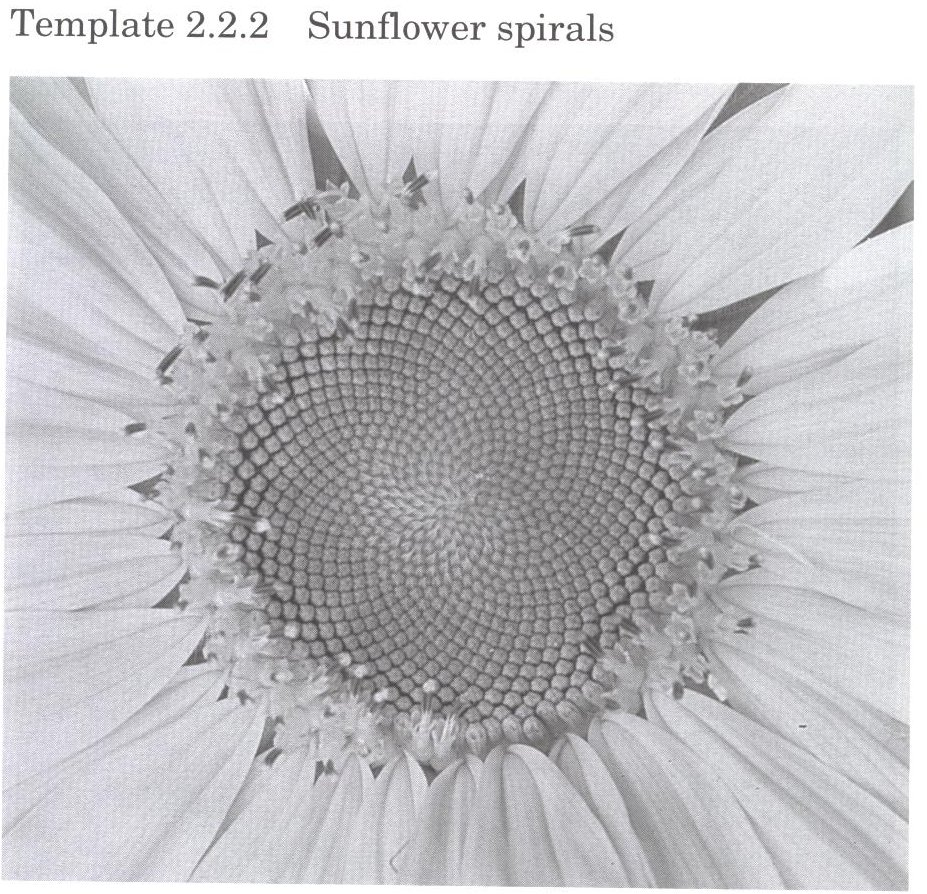#### Discovering the Fibonacci pattern through mathematical explorations

##### Honeycomb Walk

A mathematical bee, starting in cell one of the simple honeycomb design shown in the figure wishes to stroll to another higher number cell via a path of connected cells not necessarily in numerical order, but always increasing. That is, each step of the journey must head to the right, so the bee can only move from one cell to a neighboring cell of a higher number. In how many different ways can the bee travel from cell 1 to cell 4? To cell 5? To cell 6? Can you see a pattern developing?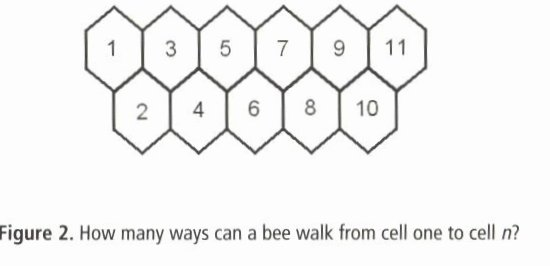##### A funny new language: ABABA

The language of “ABABA” uses only two letters: A and B. No word in the language may contain two consecutive B’s. but any string of letters avoiding two consecutive Bs is indeed a word. For example, “AABAAAAB” and “BAA” are both words, but “ABBA” is not. How many N-lettered words does ABABA posses?

If I toss a coin 3 times, in how many ways will I not get two heads in a row? What is the probability I won’t see two heads in a row? Same questions, but now in four tosses of the coin? Same question but now in five tosses of the coin? What is the relationship to the previous questions?

#### Integers as sums of Fibonacci numbers

It is really is sad that every number is not a Fibonacci number. Is there any way to express 15 as a sum of Fibonacci numbers? Is there any way to express 20 as a sum of Fibonacci numbers? Is there any way to express 25 as a sum of Fibonacci numbers?

#### Ratios of Fibonacci numbers

Make a reasonably large table of Fibonacci numbers and explore the relative sizes of two adjacent Fibonacci numbers, the bigger of the adjacent pair is not quite two times the smaller. Use your calculator to explore the ratios, and make a conjecture about what number the ratio tends toward.

#### Arithmetic and Geometric Sequences

An arithmetic sequence is a sequence of numbers of the form a, a + d, a + 2d, a + 3d, and so on. a is the initial term, and d is the common difference. A useful trick when working with arithmetic sequences is to notice that for three numbers in arithmetic sequence the middle number is the average of the other two, i.e. let the numbers in arithmetic sequence be a - d, a and a + d, then a = (1/2)((a + d)(a - d)).

A geometric sequence is a sequence of numbers of the form a, ar, ar2, ar3, and so on. a is the initial term, and r is the common ratio. Notice that if gn and gn+1 are successive terms in a geometric sequence, then gn+1/gn = r. A useful trick when working with geometric sequences is to notice that for three numbers in arithmetic sequence the square of the middle number is the product of the other two, i.e. let the numbers in arithmetic sequence be a/r, a and ar, then a2 = ((a/r)(ar)).

This section is adapted from: Instructor Resources and Adjunct Guide for the second edition of The Heart of Mathematics by E. Burger, M. Starbird, and D. Bergstrand.

### Sample Worked Problems

#### Problem 16, Page 60, Sum of Fibonacci

Choose the largest Fibonacci number less than the given number, take it out, and continue with the remainder: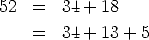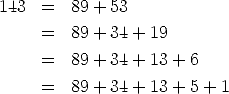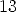is a Fibonacci number already, so no further decomposition is necessary.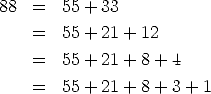#### Problem 27, page 61, Finding Factors

The first ten even index Fibonacci numbers are 1, 3, 8, 21, 55, 144, 377, 987, 2584, 6765. The only way to factor the first is F2 = 1 = 1 . 1. Likewise, the only way to factor the second is F4 = 3 = 3 . 1. There are two ways to factor the third, namely F6 = 8 = 1 . 8, 8 = 2 . 4. There are likewise two ways to factor the fourth, F8 = 21 = 1 . 21 and 21 = 3 . 7. And the fifth likewise, F10 = 55 = 1 . 55 and 55 = 5 . 11. However there are many ways to factor the next Fibonacci number F12 = 144 in the sequence. So the pattern can’t depend on an obvious factorization into two factors. Using the hint from the problem, look at the factors themselves in light of the Lucas sequence. The Lucas numbers are 2, 1, 3, 4, 7, 11, 18, 29, 47, 76, 123, 199, 322, . . . . After some experimentation, and some trial and error (it took me three attempts to get it just right, each attempt closer than the previous) we notice that F2 = F1 . L2. Next we notice that F4 = F2 . L3. Next we observe that F6 = F3 . L4, and F8 = F4 . L5. The pattern continues with 55 = F10 = F5 . L6 = 5 . 11. This is good! This pattern is subtle, but studying it for a while suggests maybe F2n = Fn . Ln+1. Test it out with the next even index Fibonacci number, the one we didn’t factor. The pattern would predict F12 = F6 . L7, or 144 = 8 . 18, which works. We seem to have it precisely. Just to extend our conjecture, test 6765 = F20 = F10 . L11 = 55 . 123. This gives great credence to our conjecture.

#### Problem 37, page 62, Too Small

Let N be a natural number which is not a Fibonacci number. Let F be the largest Fibonacci number less than N, so that we can write F < N. To be definite, suppose that F = Fn. Then let Fi and Fj be two distinct Fibonacci numbers less than F otherwise written as Fn. Since the two indices are distinct, suppose that i is the lesser of the two indices i < j. Then Fj < Fn-1 and Fi < Fn-2. We know that Fn = Fn-1 + Fn-2. Therefore, putting all this together, Fi + Fj < Fn-2 + Fn-1 = Fn = F < N. So N can’t be written as the sum of two Fibonacci numbers less than F.

### Problems to Work for Understanding

1. Solidifying Ideas: pages 57-58: 1,2,4,5,
2. New Ideas: page 61: 4,5
3. Habits of Mind: page 62: number 3